## Induced EMF

7-21-99

So far we've dealt with electricity and magnetism as separate topics. From now on we'll investigate the inter-connection between the two, starting with the concept of induced EMF. This involves generating a voltage by changing the magnetic field that passes through a coil of wire.

We'll come back and investigate this quantitatively, but for now we can just play with magnets, magnetic fields, and coils of wire. You'll be doing some more playing like this in one of the labs. There are also some coils and magnets available in the undergraduate resource room - please feel free to use them.

First, connect a coil of wire to a galvanometer, which is just a very sensitive device we can use to measure current in the coil. There is no battery or power supply, so no current should flow. Now bring a magnet close to the coil. You should notice two things:

1. If the magnet is held stationary near, or even inside, the coil, no current will flow through the coil.

2. If the magnet is moved, the galvanometer needle will deflect, showing that current is flowing through the coil. When the magnet is moved one way (say, into the coil), the needle deflects one way; when the magnet is moved the other way (say, out of the coil), the needle deflects the other way. Not only can a moving magnet cause a current to flow in the coil, the direction of the current depends on how the magnet is moved.

How can this be explained? It seems like a constant magnetic field does nothing to the coil, while a changing field causes a current to flow.

To confirm this, the magnet can be replaced with a second coil, and a current can be set up in this coil by connecting it to a battery. The second coil acts just like a bar magnet. When this coil is placed next to the first one, which is still connected to the galvanometer, nothing happens when a steady current passes through the second coil. When the current in the second coil is switched on or off, or changed in any way, however, the galvanometer responds, indicating that a current is flowing in the first coil.

You also notice one more thing. If you squeeze the first coil, changing its area, while it's sitting near a stationary magnet, the galvanometer needle moves, indicating that current is flowing through the coil.

What you can conclude from all these observations is that a changing magnetic field will produce a voltage in a coil, causing a current to flow. To be completely accurate, if the magnetic flux through a coil is changed, a voltage will be produced. This voltage is known as the induced emf.

The magnetic flux is a measure of the number of magnetic field lines passing through an area. If a loop of wire with an area A is in a magnetic field B, the magnetic flux is given by: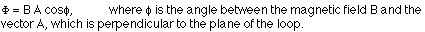If the flux changes, an emf will be induced. There are therefore three ways an emf can be induced in a loop:

1. Change the magnetic field
2. Change the area of the loop
3. Change the angle between the field and the loop

We'll move from the qualitative investigation of induced emf to the quantitative picture. As we have learned, an emf can be induced in a coil if the magnetic flux through the coil is changed. It also makes a difference how fast the change is; a quick change induces more emf than a gradual change. This is summarized in Faraday's law of induction. The induced emf in a coil of N loops produced by a change in flux in a certain time interval is given by: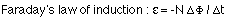Recalling that the flux through a loop of area A is given by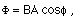The negative sign in Faraday's law comes from the fact that the emf induced in the coil acts to oppose any change in the magnetic flux. This is summarized in Lenz's law.

Lenz's law: The induced emf generates a current that sets up a magnetic field which acts to oppose the change in magnetic flux.

Another way of stating Lenz's law is to say that coils and loops like to maintain the status quo (i.e., they don't like change). If a coil has zero magnetic flux, when a magnet is brought close then, while the flux is changing, the coil will set up its own magnetic field that points opposite to the field from the magnet. On the other hand, a coil with a particular flux from an external magnetic field will set up its own magnetic field in an attempt to maintain the flux at a constant level if the external field (and therefore flux) is changed.

### An example

Consider a flat square coil with N = 5 loops. The coil is 20 cm on each side, and has a magnetic field of 0.3 T passing through it. The plane of the coil is perpendicular to the magnetic field: the field points out of the page.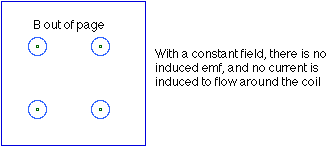(a) If nothing is changed, what is the induced emf?

There is only an induced emf when the magnetic flux changes, and while the change is taking place. If nothing changes, the induced emf is zero.

(b) The magnetic field is increased uniformly from 0.3 T to 0.8 T in 1.0 seconds. While the change is taking place, what is the induced emf in the coil?

Probably the most straight-forward way to approach this is to calculate the initial and final magnetic flux through the coil.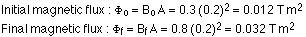The induced emf is then: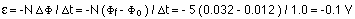(c) While the magnetic field is changing, the emf induced in the coil causes a current to flow. Does the current flow clockwise or counter-clockwise around the coil?

To answer this, apply Lenz's law, as well as the right-hand rule. While the magnetic field is being changed, the magnetic flux is being increased out of the page. According to Lenz's law, the emf induced in the loop by this changing flux produces a current that sets up a field opposing the change. The field set up by the current in the coil, then, points into the page, opposite to the direction of the increase in flux. To produce a field into the page, the current must flow clockwise around the loop. This can be found from the right hand rule.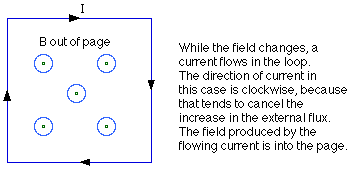One way to apply the rule is this. Point the thumb on your right hand in the direction of the required field, into the page in this case. If you curl your fingers, they curl in the direction the current flows around the loop - clockwise.

### Motional emf

Let's say you have a metal rod, and decide to connect that to your galvanometer. If the rod is stationary in a magnetic field, nothing happens. If you move the rod through the field, however, an emf is induced between the ends of the rod causing current to flow. This is because when you move the metal rod through the field, you are moving all the electrons in the rod. These moving charges are deflected by the field toward one end of the rod, creating a potential difference. This is known as motional emf. Motional emf can even be measured on airplanes. As the plane flies through the Earth's magnetic field, an emf is induced between the wingtips.

Motional emf is largest when the direction of motion of the piece of metal is perpendicular to the rod and perpendicular to the magnetic field. When this is true, the motional emf is proportional to the speed of the rod, the length (L) of the rod, and the magnetic field: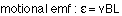If the metal rod is part of a complete circuit, the induced emf will cause a current to flow. Because it's in a magnetic field, the rod experiences a force because of the interaction between the field and the current. This force always acts to oppose the motion of the rod.

When we looked at DC motors, we saw how the force exerted on a current flowing around a coil in a magnetic field can produce rotation, transforming electrical energy to mechanical energy. Motional emf is a good example of how mechanical energy, energy associated with motion, can be transformed to electrical energy.